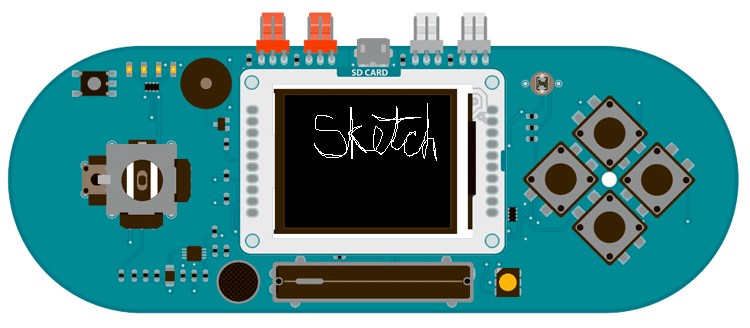Examples > TFT

This example for the Arduino TFT screen and Esplora draws a white line on the screen, based on the position of the joystick. To clear the screen, shake the Esplora. The values from the accelerometer will determine if the screen should be cleared or not.

### Hardware Required

• Arduino Esplora
• Arduino TFT screen

### CircuitAttach the screen to the socket on your Esplora, with the label "SD Card" facing up.

### Code

To use the screen you must first include the SPI and TFT libraries. Don't forget to include the Esplora library as well.

#include <Esplora.h>
#include <TFT.h>
#include <SPI.h>

Set up the cursor's x & y position. In the example, it starts in the center of the screen; determined by dividing the height and width of the screen by 2.

int xPos = EsploraTFT.width()/2;
int yPos = EsploraTFT.height()/2;

In `setup()`, initialize the display and clear the screen's background.

void setup() {
EsploraTFT.begin();
EsploraTFT.background(0,0,0);
}

Read the values of the joystick and map it to a smaller number. It's possible that your joystick doesn't read 0 when it is in the center position. To make sure the cursor only moves when the joystick is intentionally moved, use an if statement to check if it's position is inside a threshold (in this case -10 to 10). If it's within that range, son;t update the cursor position. However, if it is outside of that, move the cursor in the appropriate direction.

void loop()
{

if (xAxis<10 && xAxis>-10){
xPos=xPos;
}
else{
xPos = xPos + (map(xAxis, -512, 512, 2, -2));
}
if (yAxis<10 && yAxis>-10){
yAxis=yAxis;
}
else{
yPos = yPos + (map(yAxis, -512, 512, -2, 2));
}

You'll want to keep the cursor from moving offscreen with a few `if()` statements before you draw the point.

if(xPos > 159){
(xPos = 159);
}

if(xPos < 0){
(xPos = 0);
}
if(yPos > 127){
(yPos = 127);
}

if(yPos < 0){
(yPos = 0);
}

EsploraTFT.stroke(255,255,255);
EsploraTFT.point(xPos,yPos);

Finally, check the value of the accelerometer. If the value of the x or y axis is over the threshold you determine, clear the screen with `background()`.

EsploraTFT.background(0,0,0);
}

delay(33);
}

The complete sketch is below :

/*

This example for the Arduino TFT and Esplora draws
a white line on the screen, based on the position
of the joystick. To clear the screen, shake the
Esplora, using the values from the accelerometer.

This example code is in the public domain.

Created 15 April 2013 by Scott Fitzgerald

*/

#include <Esplora.h>
#include <TFT.h>  // Arduino LCD library
#include <SPI.h>

// initial position of the cursor
int xPos = EsploraTFT.width() / 2;
int yPos = EsploraTFT.height() / 2;

void setup() {
// initialize the display
EsploraTFT.begin();

// clear the background
EsploraTFT.background(0, 0, 0);
}

void loop() {

// update the position of the line
// depending on the position of the joystick
if (xAxis < 10 && xAxis > -10) {
xPos = xPos;
} else {
xPos = xPos + (map(xAxis, -512, 512, 2, -2));
}
if (yAxis < 10 && yAxis > -10) {
yAxis = yAxis;
} else {
yPos = yPos + (map(yAxis, -512, 512, -2, 2));
}

// don't let the point go past the screen edges
if (xPos > 159) {
(xPos = 159);
}

if (xPos < 0) {
(xPos = 0);
}
if (yPos > 127) {
(yPos = 127);
}

if (yPos < 0) {
(yPos = 0);
}

// draw the point
EsploraTFT.stroke(255, 255, 255);
EsploraTFT.point(xPos, yPos);

// check the accelerometer values and clear
// the screen if it is being shaken